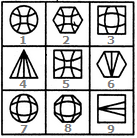# Non Verbal Reasoning - Grouping of Images - Discussion

### Discussion :: Grouping of Images - Section 1 (Q.No.8)

In each of the following questions, group the given figures into three classes using each figure only once.

8.

Group the given figures into three classes using each figure only once.[A]. 1,2,5 ; 3,7,8 ; 4,6,9 [B]. 1,7,2 ; 3,9,6 ; 4,5,8 [C]. 2,3,8 ; 4,6,9 ; 1,5,7 [D]. 5,6,9 ; 3,4,1 ; 2,7,8

Explanation:

1, 2, 5 are figures that have patterns formed from four lines curved in a concave direction.

3, 7, 8 are figures that have patterns formed from four lines curved in a convex direction.

4, 6, 9 are figures that have patterns formed from these straight lines.